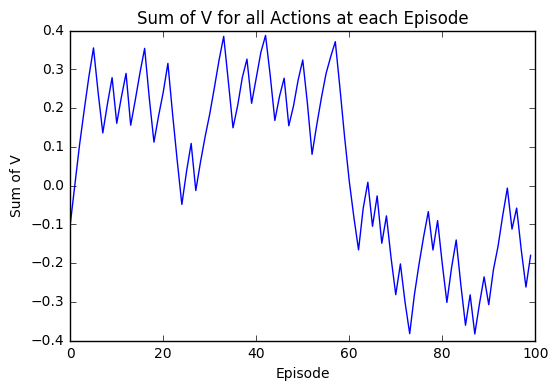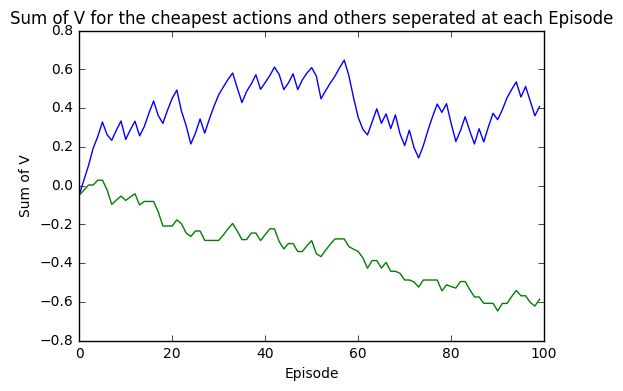# Reinforcement Learning for Meal Planning based on Meeting a Set Budget and Personal Preferences¶

## Aim¶

When food shopping, there are many different products for the same ingredient to choose from in supermarkets. Some are less expensive, others are of higher quality. I would like to create a model that, for the required ingredients, can select the optimal products required to make a meal that is both:

1. Within my budget
2. Meets my personal preferences

To do this, I will first build a very simple model that can recommend the products that are below my budgets before introducing my preferences.

The reason we use a model is so that we could, in theory, scale the problem to consider more and more ingredients and products that would cause the problemt to then be beyond the possibility of any mental calculations.

## Method¶

To achieve this, I will be bulding a simple reinforcement learning model and use Montel Carlo learning to find the optimal combination of products.

First, let us formally define the parts of our model as a Markov Decision Process:

• We have a finite number of ingredients required to make any meal and are considered to be our States
• There are the finite possible products for each ingredient and are therefore the Actions of each state
• Our preferences become the Individual Rewards for selecting each product, we will cover this in more detail later

Monte Carlo learning takes the combined the quality of each step towards reaching an end goal and requires that, in order to assess the quality of any step, we must wait and see the outcome of the whole combination.

Monte Carlo is often avoided due to the time required to go through the whole process before being able to learn. However, in our problem it is required as our final check when establishing whether the combination of products selected is good or bad is to add up the real cost of those selected and check whether or not this is below or above our budget. Futhermore, at least at this stage, we will not be considering more than a few ingredients and so the time taken is not significant in this regard.

## Sample Data¶

For this demonstration, I have created some sample data for a meal where we have 4 ingredients and 9 products, as shown in the feature image.

We need to select one product for each ingredient in the meal.

This means we have 2 x 2 x 2 x 3 = 24 possible selections of products for the 4 ingredients.

I have also included the real cost for each product and V_0.

V_0 is simply the initial quality of each product to meet our requirements and we set this to 0 for each.

In :
import pandas as pd
import numpy as np
import matplotlib.pyplot as plt

import warnings
warnings.filterwarnings('ignore')

import time

In :
data = pd.read_csv('C:\\Users\\kr015\\Documents\\Machine Learning\Meal Plan\\Final\\SampleData.csv')

In :
data

Out:
Ingredient Product QMerged_label Real_Cost V_0
0 1 1 11 10 0
1 1 2 12 6 0
2 2 1 21 8 0
3 2 2 22 11 0
4 3 1 31 3 0
5 3 2 32 7 0
6 4 1 41 8 0
7 4 2 42 5 0
8 4 3 43 1 0

## Applying the Model in Theory¶

For now, I will not introduce any individual rewards for the procuts. Intead, I will simply focus on whether the combination of products selected is below our budget or not. This outcome is defined as the Terminal Reward of our problem.

For example, say we have a budget of £30, then the choice:

$$a1 \rightarrow b1 \rightarrow c1 \rightarrow d1$$

Then the real cost of this selection is:

$$£10 + £8 + £3 + £8 = £29 < £30$$

And therefore, our terminal reward is:

$$R_T = +1$$

Whereas,

$$a2 \rightarrow b2 \rightarrow c2 \rightarrow d1$$

Then the real cost of this selection is:

$$£6 + £11 + £7 + £8 = £32 > £30$$

And therefore, our terminal reward is:

$$R_T = -1$$

For now, we are simply telling our model whether the choice is good or bad and will observe what this does to the results.

### Model Learning¶

So how does our model actually learn? In short, we get our model to try out lots of combinations of proucts and at the end of each tell it whether its choice was good or bad. Over time, it will recognise that some products generally lead to getting a good outcome while others do not.

What we end up creating are values for how good each product is, denoted V(a). We have already introduced the initial V(a) for each product but how do we reach go from these initial values to actually being able to make a decision?

For this, we need an Update Rule. This tells the model, after each time it has presented its choice of products and we have told it whether it’s selection is good or bad, how to add this to our initial values.

Our update rule is as follows:

$$V(a) \leftarrow V(a) + \alpha*(G – V(a))$$

This may look unusual at first but in words we are simply updating the value of any action, V(a), by an amount that is either a little more if the outcome was good or a little less if the outcome was bad.

G is the Return and is simply to total reward obtained. Currently in our example, this is simply the terminal reward (+1 or -1 accordingly). We will reintroduce this later when we include individual product rewards.

Alpha, $\alpha$, is the Learning Rate and we will demonstrate how this effects the results more later but just for now, the simple explination is: “The learning rate determines to what extent newly acquired information overrides old information. A factor of 0 makes the agent learn nothing, while a factor of 1 makes the agent consider only the most recent information.” (https://en.wikipedia.org/wiki/Q-learning)

### Small Demo of Updating Values¶

So how do we actually use this with our model?

Let us start with a table that has each product and its initial V_0(a):

Product V_0(a)
a1 0
a2 0
b1 0
b2 0
c1 0
c2 0
d1 0
d2 0
d3 0

We now pick a random selection of products, each cobination is known as an episode. We also set $\alpha = 0.5$ for now just for simpicity in the calculations.

e.g.

$$a1 \rightarrow b1 \rightarrow c1 \rightarrow d1$$

Provides:

$$Total Cost = £29 < £30$$

Therefore:

$$R_T = +1$$

Now applying our update rule to a1:

$$V_1(a1) <- V_0(a1) + \alpha*( G – V_0(a1))$$

$$\implies V_1(a1) <- 0 + 0.5*( 1 – 0) = 0.5$$

Therefore, all actions that lead to this positive outcome are updated as well to produced the following table with V1(a):

Product V_0(a) V_1(a)
a1 0 0.5
a2 0 0
b1 0 0.5
b2 0 0
c1 0 0.5
c2 0 0
d1 0 0.5
d2 0 0
d3 0 0

So let us pick another random episode:

$$a1 \rightarrow b2 \rightarrow c2 \rightarrow d1$$

Provides:

$$Total Cost = £36 > £30$$

Therefore:

$$R_T = -1$$

Now applying our update rule to a1:

$$V_2(a1) \leftarrow V_1(a1) + \alpha * ( G – V_1(a1))$$

$$\implies V_2(a1) \leftarrow 0.5 + 0.5*( -1 – 0.5) = 0.5 – 0.75$$

$$\implies V_2(a1) \leftarrow -0.25$$

and likewise for d1.

Whereas the updated value for b2 is:

$$V_2(b2) \leftarrow V_1(b2) + \alpha*( G – V_1(b2))$$

$$\implies V_2(b2) \leftarrow 0 + 0.5*( -1 – 0) = -0.5$$

and likewise for c2.

Therefore, we can add V2(a) to our table:

Product V_0(a) V_1(a) V_2(a)
a1 0 0.5 -0.25
a2 0 0 0
b1 0 0.5 0.5
b2 0 0 -0.5
c1 0 0.5 0.5
c2 0 0 -0.5
d1 0 0.5 -0.25
d2 0 0 0
d3 0 0 0

## Action Selection¶

You may have noticed in the demo, I have simply randomly selected the products in each episode. We could do this but using a completely random selection process may mean that some actions are not selected often enough to know whether they are good or bad.

Similarly, if we went to other way and decided to select the products greedily, i.e. to ones that currently have the best value, we may miss one that is in fact better but never given a chance. For example, if we chose the best actions from V2(a) we would get a2, b1, c1 and d2 or d3 which both provide a positive terminal reward therefore, if we used a purely greedy selection process, we would never consider any other products as these continue to provide a positiv outcome.

Instead, we implement epsilon-greedy action selection where we randomly select products with probablity $\epsilon$, and greedily select products with probability $1-\epsilon$ where:

$$0 \leq \epsilon \leq 1$$

This means that we are going reach the optimal choice of products quickly as we continue to test whether the ‘good’ products are in fact optimal but also leaves room for us to also explore other products occasionally just to make sure they aren’t as good as our current choice.

# Building and Applying our Model¶

We are now ready to build a simple model as shown in the MCModelv1 function below.

Although this seems complex, I have done nothing more than apply the methods previously discussed in such a way that we can vary the inputs and still obtain reqults. Admittedly, this was my first attempt at doing this and so my coding may not be perfectly written but should be sufficient for our requirements.

To calculate the terminal reward, we currently use the following condition to check if the total cost is less or more than our budget:

if(budget >= episode2['Real_Cost'].sum()):
Return = 1
else:
Return = -1
In :
def MCModelv1(data, alpha, e, epsilon, budget, reward):
# Define the States
Ingredients = list(set(data['Ingredient']))
# Initialise V_0
V0 = data['V_0']
data['V'] = V0
output = []
output1 = []
output2 = []
actioninfull = []
#Interate over the number of episodes specified
for e in range(0,e):

episode_run = []
#Introduce epsilon-greedy selection, we randomly select the first episode as V_0(a) = 0 for all actions
epsilon = epsilon
if e == 0:
for i in range(0,len(Ingredients)):
episode_run = np.append(episode_run,np.random.random_integers(low = 1, high = sum(1 for p in data.iloc[:, 0] if p == i+1 ), size = None))
episode_run = episode_run.astype(int)

else:
for i in range(0,len(Ingredients)):
greedyselection = np.random.random_integers(low = 1, high =10)
if greedyselection <= (epsilon)*10:
episode_run = np.append(episode_run,np.random.random_integers(low = 1, high = sum(1 for p in data.iloc[:, 0] if p == i+1 ), size = None))
else:
data_I = data[data['Ingredient'] == (i+1)]
MaxofVforI = data_I[data_I['V'] == data_I['V'].max() ]['Product']
#If multiple max values, take first
MaxofVforI = MaxofVforI.values
episode_run = np.append(episode_run, MaxofVforI)

episode_run = episode_run.astype(int)

episode = pd.DataFrame({'Ingredient' : Ingredients, 'Product': episode_run})
episode['Merged_label'] =  (episode['Ingredient']*10 + episode['Product']).astype(float)
data['QMerged_label'] = (data['QMerged_label']).astype(float)
data['Reward'] = reward
episode2 =  episode.merge(data[['QMerged_label','Real_Cost','Reward']], left_on='Merged_label',right_on='QMerged_label', how = 'inner')
data = data.drop('Reward',1)

# Calculate our terminal reward
if(budget >= episode2['Real_Cost'].sum()):
Return = 1
else:
Return = -1
episode2 = episode2.drop('Reward',1)
episode2['Return'] = Return

# Apply update rule to actions that were involved in obtaining terminal reward
data = data.merge(episode2[['Merged_label','Return']], left_on='QMerged_label',right_on='Merged_label', how = 'outer')
data['Return'] = data['Return'].fillna(0)
for v in range(0,len(data)):
if data.iloc[v,7] == 0:
data.iloc[v,5] = data.iloc[v,5]
else:
data.iloc[v,5]  = data.iloc[v,5]  + alpha*( (data.iloc[v,7]/len(Ingredients)) - data.iloc[v,5] )

# Output table
data = data.drop('Merged_label',1)
data = data.drop('Return',1)

# Output is the Sum of V(a) for all episodes
output  = np.append(output, data.iloc[:,-1].sum())

# Output 1 and 2 are the Sum of V(a) for for the cheapest actions and rest respectively
# I did this so we can copare how they converge whilst applying to such a small sample problem
output1 = np.append(output1, data.iloc[[1,2,4,8],-1].sum())
output2 = np.append(output2, data.iloc[[0,3,5,6,7],-1].sum())

# Ouput to optimal action from the model based on highest V(a)
action = pd.DataFrame(data.groupby('Ingredient')['V'].max())
action2 = action.merge(data, left_on = 'V',right_on = 'V', how = 'inner')
action3 = action2[['Ingredient','Product']]
action3 = action3.groupby('Ingredient')['Product'].apply(lambda x :x.iloc[np.random.randint(0, len(x))])

# Output the optimal action at each episode so we can see how this changes over time
actioninfull = np.append(actioninfull, action3)
actioninfull = actioninfull.astype(int)

# Rename for clarity
SumofV = output
SumofVForCheapest = output1
SumofVForExpensive = output2
OptimalActions = action3
ActionsSelectedinTime = actioninfull

return(SumofV, SumofVForCheapest, SumofVForExpensive, OptimalActions, data, ActionsSelectedinTime)


### We now run our model with some sample variables:¶

In :
alpha = 0.1
num_episodes = 100
epsilon = 0.5
budget = 30

# Currently not using a reward
reward = [0,0,0,0,0,0,0,0,0]

start_time = time.time()

Mdl = MCModelv1(data=data, alpha = alpha, e = num_episodes,epsilon = epsilon, budget = budget, reward = reward)

print("--- %s seconds ---" % (time.time() - start_time))

--- 3.3326752185821533 seconds ---


In our function, we have 6 outputs from the model.

• Mdl: Returns the Sum of all V(a) for each episode
• Mdl: Returns to Sum of V(a) for the cheapest products, possible to define due to the simplicity of our sample data
• Mdl: Returns the Sum of V(a) for the non-cheapest products
• Mdl: Returns the optimal actions of the final episode
• Mdl: Returns the data table with the final V(a) added for each product
• Mdl: Shows the optimal action at each epsiode

There is a lot to take away from these so let us go through each and establish what we can learn to improve our model.

#### Optimal actions of final episode¶

First, lets see what the model suggest we should select. In this run it suggests actions, or products, that have a total cost below budget which is good.

However, there is still more that we can check to help us understand what is going on.

First, we can plot the total V for all actions and we see that this is converging which is ideal. We want our model to converge so that as we try more episodes we are ‘zoning-in’ on the optimal choice of products. The reason the output converges is because we are reducing the amount it learns each time by a factor of $\alpha$, in this case 0.5. We will show later what happens if we vary this or don’t apply this at all.

We have also plotted the sum of V for the produts we know are cheapest, based on being able to assess the small sample size, and the others seperately. Again, both are converging positively although the cheaper products appear to have slightly higher values.

In :
print(Mdl)

Mdl

Ingredient
1    2
2    1
3    1
4    3
Name: Product, dtype: int64

Out:
Ingredient Product QMerged_label Real_Cost V_0 V
0 1 1 11.0 10 0 0.231234
1 1 2 12.0 6 0 0.247748
2 2 1 21.0 8 0 0.249826
3 2 2 22.0 11 0 0.215450
4 3 1 31.0 3 0 0.249917
5 3 2 32.0 7 0 0.184853
6 4 1 41.0 8 0 0.154113
7 4 2 42.0 5 0 0.188186
8 4 3 43.0 1 0 0.249826
In :
plt.plot(range(0,num_episodes), Mdl)
plt.title('Sum of V for all Actions at each Episode')
plt.xlabel('Episode')
plt.ylabel('Sum of V')
plt.show()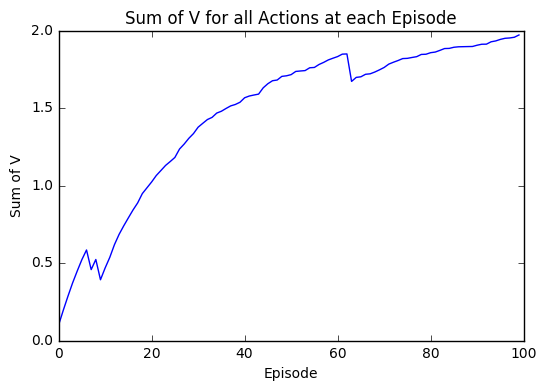In :
plt.plot(range(0,num_episodes), Mdl,range(0,num_episodes), Mdl)
plt.title('Sum of V for the cheapest actions and others seperated at each Episode')
plt.xlabel('Episode')
plt.ylabel('Sum of V')
plt.show()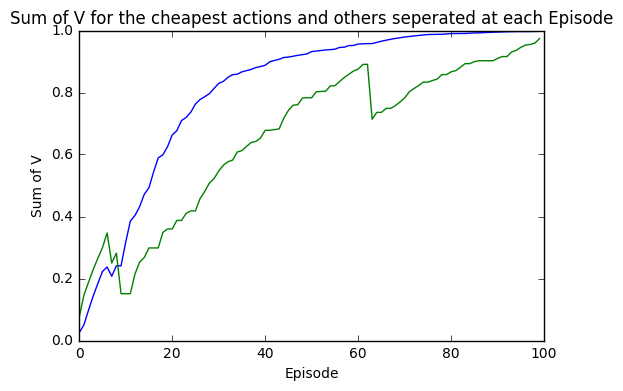#### So why is this happening and why did the model suggest the actions it did?¶

To understand that, we need to disect the suggestions made by the model at each episode and how this relates to our return.

Below, we have taken the optimal action for each state. We can see that the suggested actions do vary greatly between episodes and the model appears to decide which is wants to suggest very quickly.

Therefore, I have plotted the total cost of the suggested actions at each episode and we can see the actions vary initially then smooth out and the resulting total cost is below our budget. This helps us understand what is going on greatly.

So far, all we have told the model is to provide a selection that is below budget and it has. It has simply found a answer that is below the budget as required.

So what is the next step? Before I introduce rewards I want to demonstrate what happens if I vary some of the parameters and what we can do if we decide to change what we want our model to suggest.

In :
Ingredients = list(set(data['Ingredient']))
actions = pd.DataFrame()

for a in range(0, len(Ingredients)):
individualactions = []
for i in range(0,num_episodes):
individualactions = np.append(individualactions, Mdl[a+(i*(len(Ingredients)))])
actions[a] = individualactions
plt.plot(range(0,num_episodes), actions[a])
plt.title('Product for Ingredient: ' + str(a+1))
plt.xlabel('Episode')
plt.ylabel('Product')
plt.show()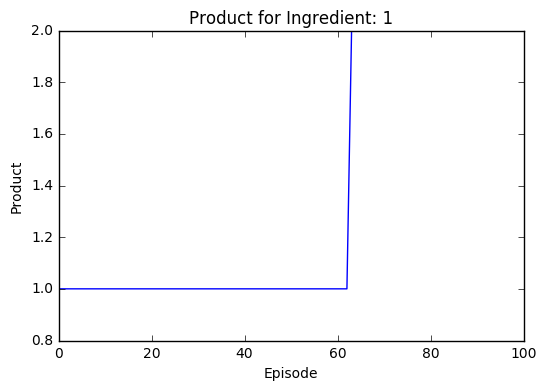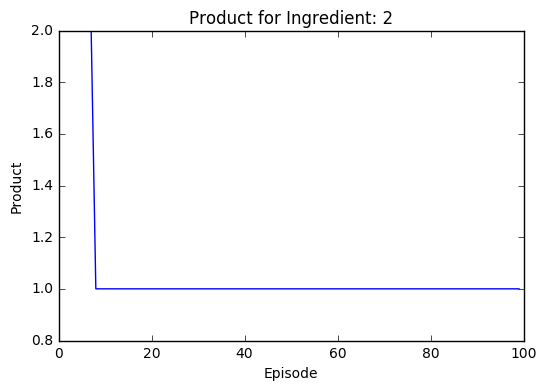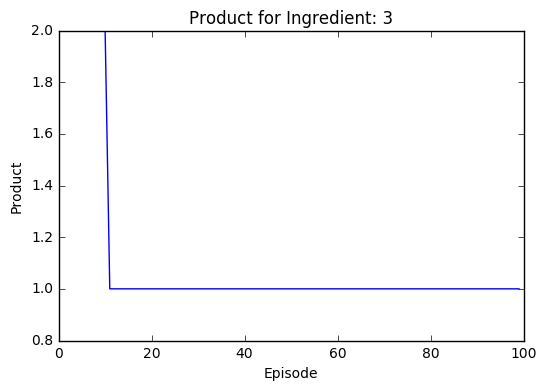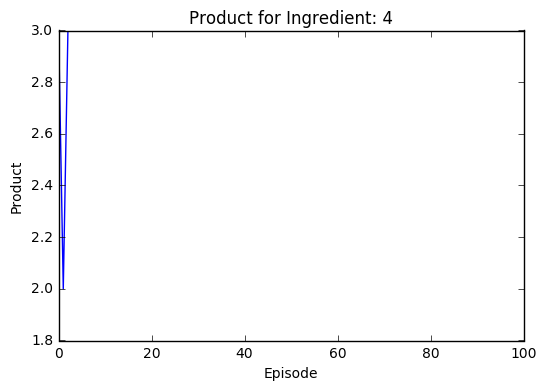In :
actions2 = actions
actions2['Product1'] = actions2.iloc[:,0]+10
actions2['Product2'] = actions2.iloc[:,1]+20
actions2['Product3'] = actions2.iloc[:,2]+30
actions2['Product4'] = actions2.iloc[:,3]+40

actions3 = actions2.merge(data[['QMerged_label','Real_Cost']],left_on = 'Product1',right_on = 'QMerged_label', how = 'left')
actions4 = actions3.merge(data[['QMerged_label','Real_Cost']],left_on = 'Product2',right_on = 'QMerged_label', how = 'left')
actions5 = actions4.merge(data[['QMerged_label','Real_Cost']],left_on = 'Product3',right_on = 'QMerged_label', how = 'left')
actions6 = actions5.merge(data[['QMerged_label','Real_Cost']],left_on = 'Product4',right_on = 'QMerged_label', how = 'left')

actions6['Total_Cost'] = actions6.iloc[:,9] + actions6.iloc[:,11] + actions6.iloc[:,13] + actions6.iloc[:,15]
actions6 = actions6.iloc[:,[0,1,2,3,-1]]

actions6 = actions6.iloc[:num_episodes]

plt.plot(range(0,num_episodes), actions6['Total_Cost'])
plt.plot([0, num_episodes], [budget, budget], 'k-', lw=2)
plt.title('Total Real Cost of Best Products at each Episode')
plt.xlabel('Episode')
plt.ylabel('Total Real Cost (£)')
plt.ylim([0,budget+10])
plt.show()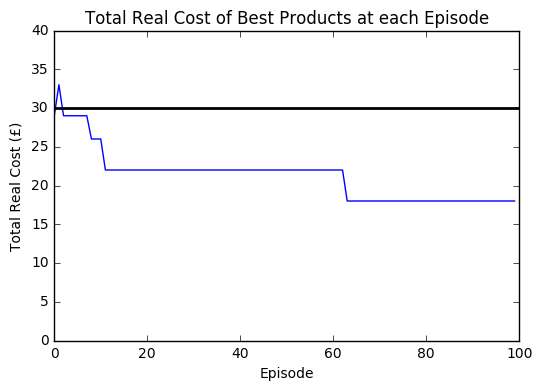## Effect of Changing Parameters and How to Change Model’s Aim¶

We have a few parameters that can be changed:

1. The Budget
2. Our learning rate, $\alpha$
3. Out action selection parameter, $\epsilon$

#### Varying Budget¶

First, lets observe what happens if we make our budget either impossibly low or high.

A budget that means we only obtain a negative reward means that we will force our V to converge negatively whereas a budget that is too high will cause our V to converge positively as all actions are continually positive.

The latter seems like what we had in our first run, a lot of the episodes lead to positive outcomes and so many combinations of products are possible and there is little distinction between the cheapest products from the rest.

If instead we consider a budget that is reasonably low given the prices of the products, we can see a trend where the cheapest products look to be converging positively and the more expensive products converging negatively. However, the smoothness of these is far from ideal, both appear to be oscillating greatly between each episode.

So what can we do the reduce the ‘spikiness’ of the outputs? This leades us onto our next parameter, alpha.

In :
##### Make budget very small
budget2 = 5

alpha2 = 0.1
num_episodes2 = 100
epsilon2 = 0.5

# Currently not using a reward
reward2 = [0,0,0,0,0,0,0,0,0]

start_time = time.time()

Mdl2 = MCModelv1(data=data, alpha = alpha2, e = num_episodes2,epsilon = epsilon2, budget = budget2, reward = reward2)

print("--- %s seconds ---" % (time.time() - start_time))

plt.plot(range(0,num_episodes2), Mdl2)
plt.title('Sum of V for all Actions at each Episode')
plt.xlabel('Episode')
plt.ylabel('Sum of V')
plt.show()

--- 2.390406608581543 seconds ---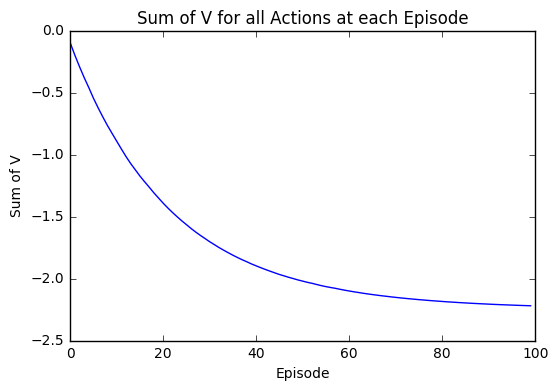In :
# Make budget very large
budget3 = 100

alpha3 = 0.1
num_episodes3 = 100
epsilon3 = 0.5

# Currently not using a reward
reward3 = [0,0,0,0,0,0,0,0,0]

start_time = time.time()

Mdl3 = MCModelv1(data=data, alpha = alpha3, e = num_episodes3,epsilon = epsilon3, budget = budget3, reward = reward3)

print("--- %s seconds ---" % (time.time() - start_time))

plt.plot(range(0,num_episodes), Mdl3)
plt.title('Sum of V for all Actions at each Episode')
plt.xlabel('Episode')
plt.ylabel('Sum of V')
plt.show()

--- 2.3504889011383057 seconds ---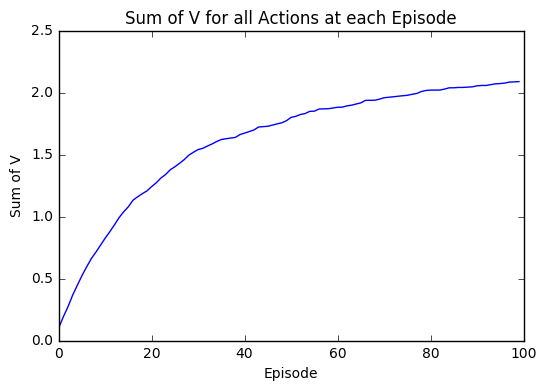In :
# Make budget reasonably small
budget4 = 23

alpha4 = 0.1
num_episodes4 = 100
epsilon4 = 0.5

# Currently not using a reward
reward4 = [0,0,0,0,0,0,0,0,0]

start_time = time.time()

Mdl4 = MCModelv1(data=data, alpha = alpha4, e = num_episodes4,epsilon = epsilon4, budget = budget4, reward = reward4)

print("--- %s seconds ---" % (time.time() - start_time))

plt.plot(range(0,num_episodes4), Mdl4)
plt.title('Sum of V for all Actions at each Episode')
plt.xlabel('Episode')
plt.ylabel('Sum of V')
plt.show()

plt.plot(range(0,num_episodes4), Mdl4,range(0,num_episodes4), Mdl4)
plt.title('Sum of V for the cheapest actions and others seperated at each Episode')
plt.xlabel('Episode')
plt.ylabel('Sum of V')
plt.show()

--- 2.2506957054138184 seconds ---